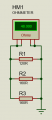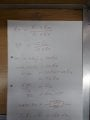Calculate Missing Resistors in a parallel circuit when given the Rtotal

rdb1

Joined Feb 6, 2019
54
So I’ve got a question that I’m working on and it’s got a table with resistors in they are connected in a parallel circuit.

R1 120 ohms R2 and R3 are empty and the Rt is 48 ohms. It maybe simple but if someone could give me an idea as to how to find the other two resistors that would be great.

djsfantasi

Joined Apr 11, 2010
8,078
What do you mean by a parallel circuit? Are all three resistors in parallel with each other? Or is one resistor in parallel with the other two in series? Which one is 120Ω?

Assuming that all three resistors are parallel with each other, mathematically, how many solutions are there?

I’d start by breaking it down into an easy problem. Disregard that two resistors are unknown for now and find the resistance of one unknown resistor in parallel with a 120Ω resistor...

WBahn

Joined Mar 31, 2012
26,398
So I’ve got a question that I’m working on and it’s got a table with resistors in they are connected in a parallel circuit.

R1 120 ohms R2 and R3 are empty and the Rt is 48 ohms. It maybe simple but if someone could give me an idea as to how to find the other two resistors that would be great.
What, exactly, are you being asked to find?

With the information you've given, all you can do is establish a relationship between R2 and R3 (such as a range of ratios that they can have).

crutschow

Joined Mar 14, 2008
28,557

rdb1

Joined Feb 6, 2019
54
We are being asked to find the value of R2 and R3. We are only give the Rtotal which is 48 ohms and R1 which is 120. They are connected in a Parallel Cirrcuit.

rdb1

Joined Feb 6, 2019
54
The title is: In the table complete the following assuming that the resistors are connected in a parallel.

LesJones

Joined Jan 8, 2017
3,661
With the information you have provided the best you can do is to get the value of the resistance of R2 in parallel with R3.

Les.

rdb1

Joined Feb 6, 2019
54
With the information you have provided the best you can do is to get the value of the resistance of R2 in parallel with R3.

Les.
How do you do that though? As that’s what I’m unsure of.

LesJones

Joined Jan 8, 2017
3,661
Read you notes (Or text book) from when you were taught about resistors in parallel. This is one of the most basic things you need to know.
NOTE we do not give the answers to homework questions. We only give hints.

Les.

rdb1

Joined Feb 6, 2019
54
Read you notes (Or text book) from when you were taught about resistors in parallel. This is one of the most basic things you need to know.
NOTE we do not give the answers to homework questions. We only give hints.

Les.
I’ve read my text book and it only shows how to work out the RTotal. Using each resistors value then finding the LCD. It doesn’t show you how to find a value of a resistor and we’ve never been shown how to do this from our notes.

DrewStupid

Joined Nov 28, 2018
64

rdb1

Joined Feb 6, 2019
54

DrewStupid

Joined Nov 28, 2018
64
Thanks, but how did you do it?
Tthrough "trial and error" ha ha
Will post calculations. Must first make them readable !

•rdb1

jayanthd

Joined Jul 4, 2015
944
$${Rt = (R1 * Rx) / (R1 + Rx)}$$

$${Rx = (Rt * R1) / (R1 - Rt)}$$

$${Rx = (48 * 120) / (120 - 48) = 80}$$

$${Rx = 80 * 2 = 160}$$ because 160 || 160 = 80

checking

$${Rt = (R1 * R2) / (R1 + R2)}$$

$${Rt = (120 * 80) / (120 + 80) = 48}$$

•rdb1

rdb1

Joined Feb 6, 2019
54
$${Rt = (R1 * Rx) / (R1 + Rx)}$$

$${Rx = (Rt * R1) / (R1 - Rt)}$$

$${Rx = (48 * 120) / (120 - 48) = 80}$$

$${Rx = 80 * 2 = 160}$$ because 160 || 160 = 80

checking

$${Rt = (R1 * R2) / (R1 + R2)}$$

$${Rt = (120 * 80) / (120 + 80) = 48}$$
That’s great, so that would work then as long as you know one resistor and the total resistance you can use the calculation to find the other resistors. I’m not sure why that’s not covered in the text book I have. But thank you I’ve made a note of that formula.

jayanthd

Joined Jul 4, 2015
944
That’s great, so that would work then as long as you know one resistor and the total resistance you can use the calculation to find the other resistors. I’m not sure why that’s not covered in the text book I have. But thank you I’ve made a note of that formula.
There was one mistake. Fixed it.

$${Rx = R2 || R3}$$

$${Rt = (R1 * Rx) / (R1 + Rx)}$$

$${Rx = (Rt * R1) / (R1 - Rt)}$$

$${Rx = (48 * 120) / (120 - 48) = 80}$$

$${Ry = 80 * 2 = 160}$$ because 160 || 160 = 80

$${Ry = R2 = R3}$$

$${Ry = (R2 * R3) / (R2 + R3) = (160 * 160) / (160 + 160) = 80}$$

checking

$${Rt = (R1 * Rx) / (R1 + Rx)}$$

$${Rt = (120 * 80) / (120 + 80) = 48}$$Last edited:
•rdb1

rdb1

Joined Feb 6, 2019
54
There was one mistake. Fixed it.

$${Rx = R2 || R3}$$

$${Rt = (R1 * Rx) / (R1 + Rx)}$$

$${Rx = (Rt * R1) / (R1 - Rt)}$$

$${Rx = (48 * 120) / (120 - 48) = 80}$$

$${Ry = 80 * 2 = 160}$$ because 160 || 160 = 80

$${Ry = R2 = R3}$$

$${Ry = (R2 * R3) / (R2 + R3) = (160 * 160) / (160 + 160) = 80}$$

checking

$${Rt = (R1 * Rx) / (R1 + Rx)}$$

$${Rt = (120 * 80) / (120 + 80) = 48}$$

View attachment 169667

What’s the attached at the bottom? Is it a program?

jayanthd

Joined Jul 4, 2015
944
What’s the attached at the bottom? Is it a program?
Yes, It is Proteus simulator.

•rdb1

LesJones

Joined Jan 8, 2017
3,661
Hi rdb1,
Re post #10 I imagine in school you would have been taught something like 1/R = 1/R1 + 1/R2 (And 1/ any other resistors in parallel.)
All you have to do is to re arrange the equation 1R = 1R1 + 1/R2 You know R and R1 (48 ohms and 120 ohms.)
So you have 1/48 = 1/120 +1/R2 (R2 in this case is your R2 and R3 in parallel) So if we re arrange the equation 1/R2 = 1/48 - 1/120 (You will have been taught how to do this in maths.) The LCD of 48 and 120 will be 240 1/R2 = 5/240 - 2/240 so 1/R2 = 3/240
So 1 = (R2 x 3 )/240 (I an showing every step rather than combining steps.) So 240 = R2 x 3 so R2 = 240/3 = 80 ohms.

Les.

•rdb1

DrewStupid

Joined Nov 28, 2018
64
Hi rdb1,
Re post #10 I imagine in school you would have been taught something like 1/R = 1/R1 + 1/R2 (And 1/ any other resistors in parallel.)
All you have to do is to re arrange the equation 1R = 1R1 + 1/R2 You know R and R1 (48 ohms and 120 ohms.)
So you have 1/48 = 1/120 +1/R2 (R2 in this case is your R2 and R3 in parallel) So if we re arrange the equation 1/R2 = 1/48 - 1/120 (You will have been taught how to do this in maths.) The LCD of 48 and 120 will be 240 1/R2 = 5/240 - 2/240 so 1/R2 = 3/240
So 1 = (R2 x 3 )/240 (I an showing every step rather than combining steps.) So 240 = R2 x 3 so R2 = 240/3 = 80 ohms.

Les.